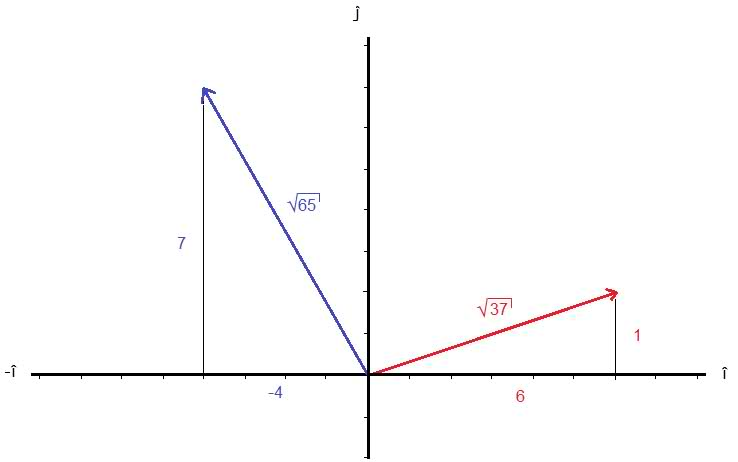# 'Simple' vector problem

## Homework Statement

We got this problem in our Physics lecture but maybe it should be in the math section. Anyway, the problem is:

If $\vec{A}+\vec{B} = 6\hat{i} + \hat{j}$, and if $\vec{A}-\vec{B} = -4\hat{i} + 7\hat{j}$, what is the magnitude of $\vec{A}$?

A) 3.0
B) 4.1
C) 5.4
D) 5.8
E) 8.2

## The Attempt at a Solution

I drew them out, but other than filling in the angles I'm not quite sure what to do next! I'd appreciate any hints on which direction to goBruceW
Homework Helper
I guess you could do it geometrically. But there is a faster way. Think of your two equations, and how you could rearrange them to get $\vec{A}$

I guess you could do it geometrically. But there is a faster way. Think of your two equations, and how you could rearrange them to get $\vec{A}$

Ohh, I didn't think of solving them as equations. The answer is 2i + 8j which = 8.2? :)

BruceW
Homework Helper
Not quite. When you add both equations together, you get: 2 $\vec{A}$ = 2i + 8j So you need to divide by 2 to get $\vec{A}$

Not quite. When you add both equations together, you get: 2 $\vec{A}$ = 2i + 8j So you need to divide by 2 to get $\vec{A}$

I need to stop making stupid mistakes like that! I see the answer is i + 4j = 4.1 now, thank you

BruceW
Homework Helper
yeah, no worries!

gneill
Mentor
To check your result graphically, draw a line segment between the tips of the two vectors you've drawn (A+B and A-B). Bisect that line segment and call the midpoint point C. Draw a vector from the origin to point C. That'll be vector A. The line segment from C to the tip of the A+B vector will be vector B. The negative of B is the line segment from C to the tip of the A-B vector.